Next: Electric Fields Up: Electricity Previous: Induced Electric Charge

## Coulomb's Law

The first precise measurement of the force between two electric charges was performed by the French scientist Charles-Augustin de Coulomb in 1788. Coulomb concluded that:
The electrical force between two charges at rest is directly proportional to the product of the charges, and inversely proportional to the square of the distance between the charges
This law of force is nowadays known as Coulomb's law. Incidentally, an electrical force exerted between two stationary charges is known as an electrostatic force. In algebraic form, Coulomb's law is written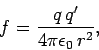(54)

where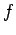is the magnitude of the force,and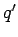are the magnitudes of the two charges (with the appropriate signs), andis the distance between the two charges. The force is repulsive if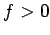, and attractive if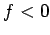. The universal constant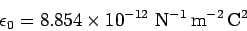(55)

is called the permittivity of free space or the permittivity of the vacuum. We can also write Coulomb's law in the form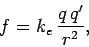(56)

where the constant of proportionality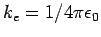takes the value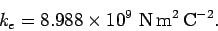(57)

Coulomb's law has an analogous form to Newton's law of gravitation,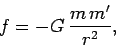(58)

with electric charge playing the role of mass. One major difference between the two laws is the sign of the force. The electrostatic force between two like charges is repulsive (i.e.,), whereas that between two unlike charges is attractive (i.e.,). On the other hand, the gravitational force between two masses is always attractive (since there is no such thing as a negative mass). Another major difference is the relative magnitude of the two forces. For instance, the electrostatic repulsion between two electrons is approximately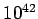times larger than the corresponding gravitational attraction.

The electrostatic force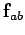exerted by a charge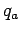on a second charge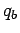, located a distancefrom the first charge, has the magnitude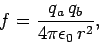(59)

and is directed radially away from the first charge if, and radially towards it if. The force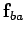exerted by the second charge on the first is equal and opposite to, so that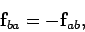(60)

in accordance with Newton's third law of motion.

Suppose that we have three point charges,,, and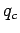. It turns out that electrostatic forces are superposable. That is, the forceexerted byonis completely unaffected by the presence of. Likewise, the force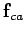exerted byonis unaffected by the presence of. Thus, the net force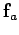acting onis the resultant of these two forces: i.e.,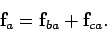(61)

This rule can be generalized in a straightforward manner to the case where there are more than three point charges.Next: Electric Fields Up: Electricity Previous: Induced Electric Charge
Richard Fitzpatrick 2007-07-14